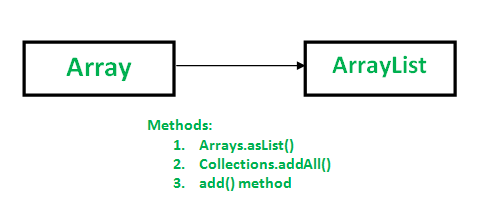Open In App

# Conversion of Array To ArrayList in Java

Following methods can be used for converting Array To ArrayList:Method 1: Using Arrays.asList() method

``` Syntax: public static List asList(T... a)
// Returns a fixed-size List as of size of given array.
// Element Type of List is of same as type of array element type.
// It returns an List containing all of the elements in this
// array in the same order.
// T represents generics ```

Note that the there is an array parameter and List return value.

• Returns a fixed-size list backed by the specified array.
• The returned list is serializable and implements RandomAccess.
• Since returned List is fixed-size therefore we can’t add more element in it, but we can replace existing element with new element using set(index, new Element) method defined in ArrayList class.

## Java

 `// Java program to demonstrate conversion of``// Array to ArrayList of fixed-size.``import` `java.util.*;` `class` `GFG``{``    ``public` `static` `void` `main (String[] args)``    ``{``        ``String[] geeks = {``"Rahul"``, ``"Utkarsh"``,``                          ``"Shubham"``, ``"Neelam"``};` `        ``// Conversion of array to ArrayList``        ``// using Arrays.asList``        ``List al = Arrays.asList(geeks);` `        ``System.out.println(al);``    ``}``}`

Output:

`[Rahul, Utkarsh, Shubham, Neelam]`

What if we add more elements to the converted list?
Since returned List is fixed-size List, we can’t add more element(s). An attempt of adding more elements would cause UnsupportedOperationException.
Consider the following example.

## Java

 `// Java program to demonstrate error``// if we add more element(s) to``// a fixed-size List.``import` `java.util.*;` `class` `GFG``{``    ``public` `static` `void` `main (String[] args)``    ``{` `        ``String[] geeks = {``"Rahul"``, ``"Utkarsh"``,``                          ``"Shubham"``, ``"Neelam"``};` `        ``// Conversion of array to ArrayList``        ``// using Arrays.asList``        ``List al = Arrays.asList(geeks);``        ``System.out.println(al);` `        ``// Adding some more values to the List.``        ``al.add(``"Shashank"``);``        ``al.add(``"Nishant"``);` `        ``System.out.println(al);``    ``}``}`

Output:

`[Rahul, Utkarsh, Shubham, Neelam]`

Runtime error

```Exception in thread "main" java.lang.UnsupportedOperationException
at GFG.main(File.java:16)```

It is therefore recommended to create new ArrayList and pass Arrays.asList(array reference) as an argument to it (i.e. as an constructor argument of ArrayList).
Consider the following example:

## Java

 `// Java program to demonstrate how to add``// one or more element(s) to returned``// resizable List.``import` `java.util.*;` `class` `GFG``{``    ``public` `static` `void` `main (String[] args)``    ``{``        ``String[] geeks = {``"Rahul"``, ``"Utkarsh"``,``                          ``"Shubham"``, ``"Neelam"``};` `        ``List al =``            ``new` `ArrayList(Arrays.asList(geeks));``        ``System.out.println(al);` `        ``// Adding some more values to the List.``        ``al.add(``"Shashank"``);``        ``al.add(``"Nishant"``);` `        ``System.out.println(``"\nArrayList After adding two"` `+``                           ``" more Geeks: "``);``        ``System.out.println(al);``    ``}``}`

```[Rahul, Utkarsh, Shubham, Neelam]

ArrayList After adding two more Geeks:
[Rahul, Utkarsh, Shubham, Neelam, Nishant, Shashank]```

&nbap;

```Syntax: public static  boolean addAll(Collection c, T... a)
// Adds all of the specified elements to the specified collection.
// Elements to be added may be specified individually or as an array.
// T is generics```

Note that there is a collection parameter c into which elements to be inserted and array parameter a contains the elements to insert into c
Return type is boolean type. It returns true if the collection changed as a result of the call.
It throws UnsupportedOperationException if collection c does not support add method and throws IllegalArgumentException if some aspect of a value in elements(or elements of array) prevents it from being added to collection c.
Consider the following example:

## Java

 `// Java program to demonstrate how to``// add all elements of array to arrayList.``import` `java.util.*;` `class` `GFG``{``    ``public` `static` `void` `main (String[] args)``    ``{``        ``String[] geeks = {``"Rahul"``, ``"Utkarsh"``,``                         ``"Shubham"``, ``"Neelam"``};` `        ``List al = ``new` `ArrayList();` `        ``// adding elements of array to arrayList.``        ``Collections.addAll(al, geeks);` `        ``System.out.println(al);``    ``}``}`

Output :

`[Rahul, Utkarsh, Shubham, Neelam]`

Note : If the specified collection or specified array is null then it throw NullpointerException.

## Java

 `// Adding null to a list``import` `java.util.*;` `class` `GFG``{``    ``public` `static` `void` `main (String[] args)``    ``{``        ``String[] geeks = {``"Rahul"``, ``"Utkarsh"``,``                         ``"Shubham"``, ``"Neelam"``};` `        ``List al = ``new` `ArrayList();``        ``Collections.addAll(``null``, geeks);` `        ``System.out.println(al);``    ``}``}`

Adding null to the end of list

## Java

 `import` `java.util.*;` `class` `GFG``{``    ``public` `static` `void` `main (String[] args)``    ``{``        ``String[] geeks = {``"Rahul"``, ``"Utkarsh"``,``                          ``"Shubham"``, ``"Neelam"``};` `        ``List al = ``new` `ArrayList();``        ``Collections.addAll(al, ``null``);` `        ``System.out.println(al);``    ``}``}`

RunTime Error

```Exception in thread "main" java.lang.NullPointerException
at GFG.main(File.java:11)```

Method 3: Using Manual method to convert Array using add() method

We can use this method if we don’t want to use java in built method(s). This is a manual method of adding all array’s elements to List.

```Syntax: public boolean add(Object obj)
// Appends the specified element to the end of this list.
// Returns true.```

## Java

 `// Java program to convert a ArrayList to``// an array using add() in a loop.``import` `java.util.*;` `class` `GFG``{``    ``public` `static` `void` `main (String[] args)``    ``{``        ``String[] geeks = {``"Rahul"``, ``"Utkarsh"``,``                          ``"Shubham"``, ``"Neelam"``};` `        ``List al = ``new` `ArrayList();` `        ``// Array to ArrayList Conversion``        ``for` `(String geek : geeks)``            ``al.add(geek);` `        ``System.out.println(al);``    ``}``}`

Output:

`[Rahul, Utkarsh, Shubham, Neelam]`

Related Article: ArrayList to Array Conversion
This article is contributed by Nitsdheerendra. If you like GeeksforGeeks and would like to contribute, you can also write an article using write.geeksforgeeks.org or mail your article to review-team@geeksforgeeks.org. See your article appearing on the GeeksforGeeks main page and help other Geeks.